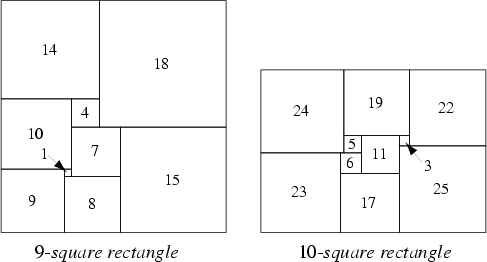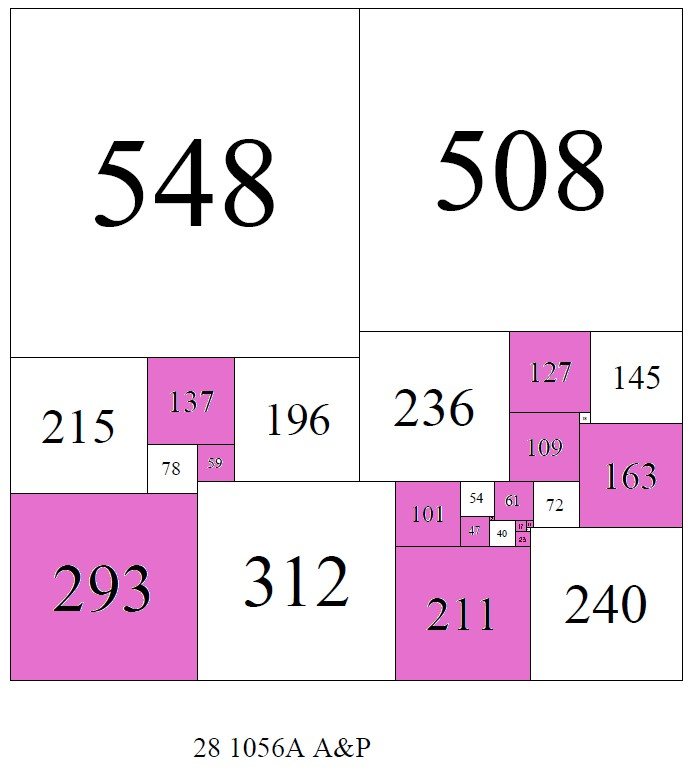Problems & Puzzles: Problems

Problem 48.  Prime Simple Perfect Squared Rectangles

More than a puzzle this is a tribute to the men that have worked in this beautiful arithmetical & geometrical field. This tribute goes mainly to Duijvestjin, Bouwkamp and the charming site/catalog from Stuart Anderson. If some of you delight the issues lovely crafted by them is enough for this puzzle. If you can get some results for the questions posed here, the better.

It's known that all numbers greater than 188 can be expressed as the arithmetic sum of at most five distinct squares; below 188, there are 31 numbers that can not be expressed as a sum of distinct squares (Sloane's A001422) and only 124 and 188 require the sum of six distinct squares.

Pebody knows (Nov. 2003) that "All numbers smaller than 100000 can be written - but greater than 17163 - as the sum of at most 16 distinct squared primes" so it's conjectured that no number will ever need to sum 17 squared primes.

But what if we change from arithmetic square decomposition of a number to geometric dissection of rectangles into geometric squares?

Here we will ask for the geometrical dissection of a given rectangle/square into several squares.

Examples:We will call the dissection to be perfect if all the squares are distinct. Otherwise the dissection is called imperfect. We will call the dissection to be simple if in doing so we do not produce two or more smaller rectangles. Otherwise the dissection is called compound.

Now is known that a simple perfect squared rectangle (spsr) uses no less than 9 squares [1925, Moron; 1940, Reichert and Toepkin] and that a simple perfect squared square (spss) uses no less than 21 squares [1978 by A. J. W. Duijvestijn].

But what about if we ask that the squares used have prime side dimension? Is there any spsr or spss having only prime squares?

For example the 10-squares rectangle shown above has 6/10 prime-squares. Using the Bouwkamp notation this rectangle is described the following way (prime are in blue):

(24,19,22)(5,11,3)(25)(23,6)(17), primes =6/10 =60%

"In this notation, brackets are used to group adjacent squares with flush tops, and then the groups are sequentially placed in the highest (and leftmost) possible slots"

I (CR) have analyzed the Bouwkamp codes of all simple perfect squared rectangles of orders from 9 to 16, and also the Bouwkamp codes of all perfect squared squares of orders from 21 to 24 (and some of orders from 25 to 27.) - the most of them produced by Duijvestjin or Bouwkamp -  codes available in the David Moews's site, and (if I made no mistake) I have found only one better prime-spsr:

(179,163)(24,139)(123,43,13)(7,17)(11,2)(9)(19,1)(18)(80), primes = 10/16=62.5%

BTW, the best prime-spss found in these files is this one:

(235,139,151)(127,12)(163)(125,79,31)(110,48)(46,14,19)(211)(9,5)(4,20)(13)(10,3)(23)(165,16)(149),
primes = 13/26 = 50.0%

I strongly recommend to visit the extremely interesting Stuart Anderson's site, if you like to display/visualize any of the rectangles or squares coded in the Moews's files (and many others that Anderson has been collecting in his beautiful site/catalog)

Questions:

1. Are impossible the 100% prime-spsr? On the contrary find one of these.
2. Are impossible the 100%
prime-
spss? On the contrary find one of these.

In the meanwhile

3. Can you find a better prime-spsr than the reported above?
4. Can you find a better prime-
spss  than the reported above?Geoff Morley (England) reports the following good new:

The following SPSS has 50% of its elements prime:
(329,311)(131,180)(157,83,89)(77,6)(71,24)(87,68)(154,3)(151)(31,149)(19,14,23,12)(43)(5,9)(111)(32)(75).
I learnt of it in a personal communication from Jasper Dale Skinner dated 3Aug2003:

"After 13 years of trying I finally produced an SPSS of 50% odd primes. I found it a fortnight ago and didn't discover its amazing quality until today. It is 2 orders higher than Duijvestijn's lone case in (order) 26, and ever so much more difficult to produce....Just the 2 cases are known in SPSS AJD's and mine."

It is 28 0640D on Stuart Anderson's website (www.squaring.net)...

Arthur Stone proved that in a perfectly squared rectangle (or square), with at least two square elements, at least two elements have even sides. This answers questions 1 and 2. His proof is on pages 149-150 of "Squared Squares: Who's Who & What's What" by Jasper Dale Skinner, II. Published by the author in 1993. ISBN: 0963656902.

The author's current address is as given in the book: 1740 North 59th Street, Lincoln, Nebraska 68505-1123, U.S.A.

***

Later Morley added a very kind and detailed answer, on request to my question for algorithms behind these kind of solutions:

A popular account of some of the work of the four undergraduates (all now deceased) at Cambridge in 1936-1938, who discovered the connection between squared rectangles and electrical networks, is given by one of them, W.T.Tutte, in the chapter on Squaring the square in Martin Gardner's "The 2nd Scientific American Book of Mathematical Puzzles and Diversions", Simon and Schuster, 1961. Also (which I have not seen) there is W.T.Tutte "The quest of the perfect square", Amer. Math. Monthly 72, No. 2, pages 29-35 (1965).

A.J.W.Duijvestijn's method of obtaining squared rectangles and squared squares by computer is summarised very briefly in A.J.W.Duijvestijn, Simple perfect squared squares and 2 X 1 squared rectangles of order 26, Mathematics of Computation, Volume 65, Number 215, Pages 1359-1364, July 1996,

A.J.W.Duijvestijn gives a detailed explanation of his methodology in A.J.W.Duijvestijn, Electronic computation of squared rectangles, Philips Research Reports 17 (1962), pages 523-612,
and a concise explanation on pages 77-86 of Skinner's book.

Both the 1962 article and the 1993 book have over 900 lines of program source code. That did not deter Jasper Skinner and Stuart Anderson who both have experience of using Duijvestijn's methodology. I do not.
Stuart Anderson (stuart@unsound.tv) in Australia has supplied programs to others and may make them available for download from www.squaring.net.

Then there's Ian Gambini, whose algorithms seem to be more concise than Duijvestijn's but his 96-page thesis "Quant aux carres carreles" ("About squared squares"), a big (1.06MB) pdf, is in French (with an English version of the Abstract on page v):
www.lim.univ-mrs.fr/~colmer/ArchivesPublications/Gambini/carres.pdf can be found by searching for "About squared squares". I have never been in contact with Gambini (gambini@iut-gtr.univ-mrs.fr ) who lives in France but originates from Spain.

Readers should note that the algorithms described in French by Gambini in chapters 4 and 5 of his thesis were first described, in English, in reference  of his thesis: Ian Gambini, A method for cutting squares into distinct squares, Discrete Applied Mathematics, Volume 98, Issues 1-2, 30 October 1999, Pages 65-80. One way for an individual to obtain this article is to purchase it from www.sciencedirect.com .

Skinner's book and both of the above pdfs have large bibliographies.

There's a short elementary article "Squaring the Square" by Ross Honsberger at
www.math.uwaterloo.ca/navigation/ideas/articles/honsberger2/index.shtml (he seems ignorant of what squares have been discovered), and I've just spotted a 19-page document "A Computer Algorithm for Finding the Dual of a Polar Network" by Sreekar M.Shastry June 19, 2003 at

There's Chapter 34 "Electrical networks and squared squares" on pages 449-460 of J.H. van Lint & R.M. Wilson's "A Course in Combinatorics", Cambridge University Press (England), 1992 (ISBN 0 521 41057 6 hardback, ISBN 0 521 42260 4 paperback) but it does not give any algorithms.

Buy Jasper's big book if you're interested in the history of the problem (who discovered what) and would like all the PSSs of orders 21-25 and many of orders 26-30 illustrated with 6 per page. It also illustrates transformation techniques whereby, for example, squares and L-shapes in one squared square can sometimes be moved around or added to to produce another square sometimes with a different number of elements.

Completely different transformation techniques are much more widely applicable to squares dissected into different right-angled isosceles triangles. Such dissections have been the main reason Jasper and I have been in frequent contact over the last two years.

***

Stuart Anderson wrote:

I have found an example of a squared rectangle 260 x 258, (SPSR) order 17 with 64.71% (11/17) prime elements, which is an improvement on the one found by yourself, (179,163)(24,139)(123,43,13)(7,17)(11,2)(9)(19,1)(18)(80), primes = 10/16=62.5%.

The bouwkampcode for the 260 x 258, (SPSR) is
(151,109)(43,66)(107,37,6,1)(5,16,23)(11)(20,7)(13,83)(70)

A new version of squaring.net will be launched soon (a much more web standard compliant site)

***

Stuart wrote again (Nov 2010):

I have recently done some more research into squared squares and have
found all of them up to order 28

i have also found a new square with 50 % of the squares prime
it is square 1056B, it can found at
;http://www.squaring.net/sq/ss/spss/o28/spsso28-2.html
the prime squares are;
7 11 17 23 47 59 61 101 109 127 137 163 211 293***

Stuart Anderson wrote on October 3, 2011:

re http://www.primepuzzles.net/problems/prob_048.htm

There are actually 2 squared squares of 29 squares with more than 50%
(51.7% = 15/29) of the squares prime, both squared squares are 767 on
the side, which is also a prime, and have the same pieces arranged
differently in a small patch of the tiling. See attached pdf file)

I think these particular one were discovered sometime over the last
year by myself, Ed Pegg Jr and Stephen Johnson in our searches in
order 29.

The bouwkampcodes are almost identical, just one transposition;
29   767  767  435  332  103  229  153  127  109  149  69  40  23  206
26  101  29  183  179  39  59  11  8  20  3  5  104  9  2  7  95

29   767  767  435  332  103  229  153  127  109  149  69  40  23  206
26  101  29  183  179  39  59  8  11  20  3  5  104  2  9  7  95

the 15 prime squares inside the 767 square are; 2, 3, 5, 7, 11, 23,
29, 59, 101, 103, 109, 127, 149, 179, 229

the interesting thing is the actual distribution of primes in squared squares,
if you count the number of primes in each squared square and sum over
the whole of order 29, you get this table;

the count of prime squares in a
SPSS;   0       1       2       3       4       5               6               7               8               9       10      11      12      13      14      15      (15 is the highest
count of primes in order 29 SPSSs)
the number of SPSS with that
count;  27      77      239     494     875     1150    1275    1208    1058    761     430     200     84      18      4       2

At the left end there are 27 SPSSs with no primes, and at the other
end, only the 2  SPSSs with 15 primes (>50%).
If you graph the frequency counts in the last table, it looks like a
normal distribution  (see attached png file)

(more correctly I should say about the distribution of the number of
distinct prime factors)

The only other place I've heard about primes and the normal
distribution is the Erdos Kac Theorem - but that's a theorem about the
distribution of prime powers in factorisation of integers.!

***Records   |  Conjectures  |  Problems  |  Puzzles